Function Repository Resource:

# CalkinWilfTree

Generate a complete binary tree of positive fractions

Contributed by: Ian Ford (Wolfram Research)
 ResourceFunction["CalkinWilfTree"][n] generates a complete binary tree of the positive fractions in the Calkin-Wilf sequence down to level n.

## Details and Options

The fractions on level d are those for which the total of the terms in its continued fraction representation is d+1.
In ResourceFunction["CalkinWilfTree"][n], n can be any non-negative machine integer.
ResourceFunction["CalkinWilfTree"] takes the same options as Tree.

## Examples

### Basic Examples (1)

Generate a complete binary tree of the positive fractions in the Calkin-Wilf sequence down to level 4:

 In:=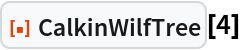Out=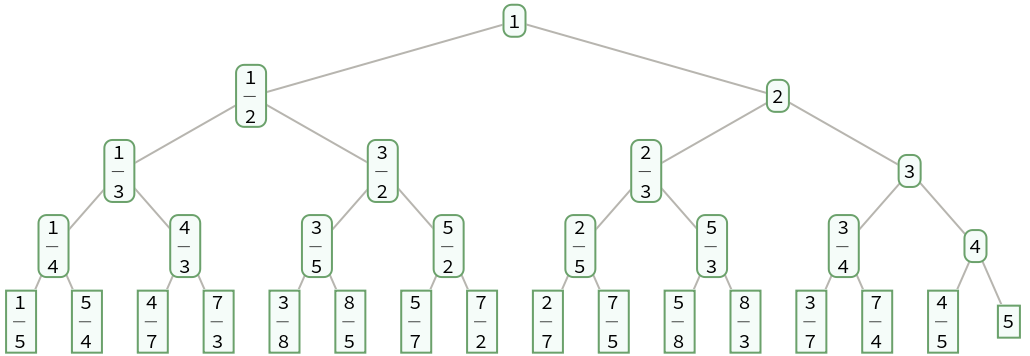### Properties and Relations (4)

A fraction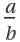has children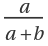and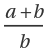:

 In:=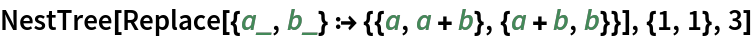Out=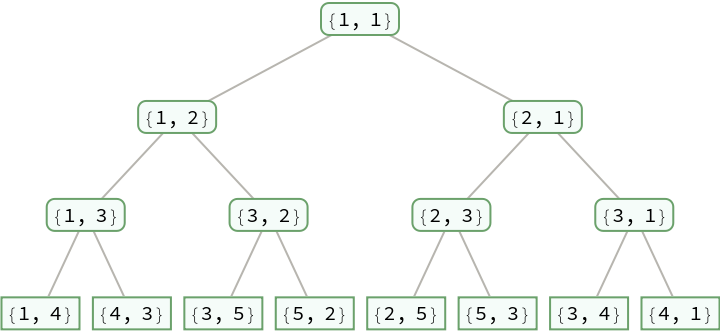In:=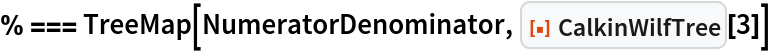Out=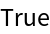The fractions on level d are those for which the total of the terms in its continued fraction representation is d+1:

 In:=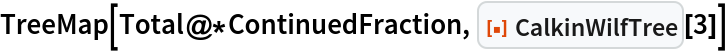Out=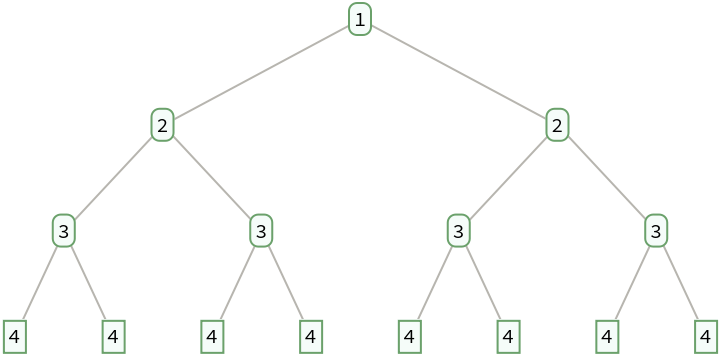The numerators of the fractions in a breadth-first traversal give Stern's diatomic series:

 In:=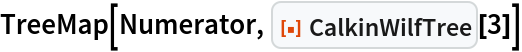Out=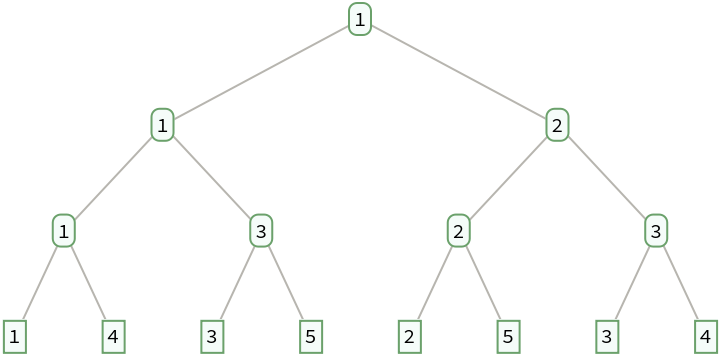In:=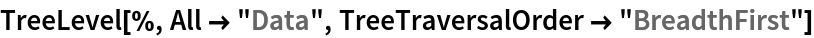Out=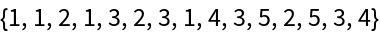The terms of Stern's diatomic series are generated by the fusc function:

 In:=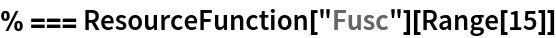Out=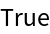Additionally, the denominators give Stern's diatomic series shifted by one:

 In:=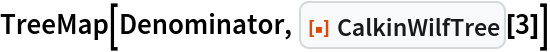Out=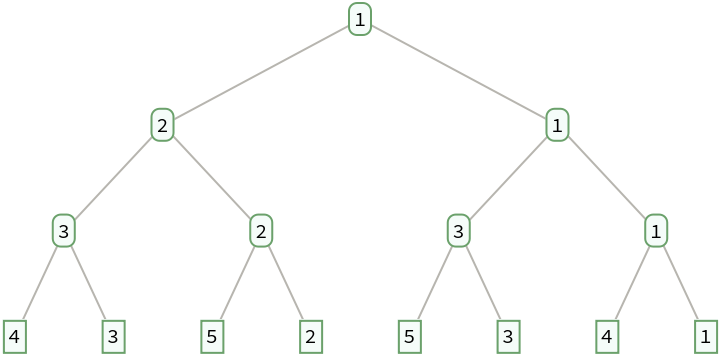In:=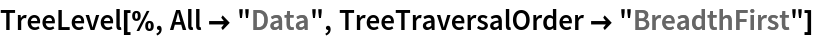Out=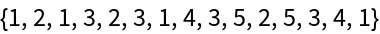If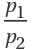,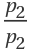, ,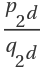are the fractions at level d, then:

 In:=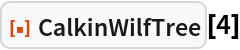Out=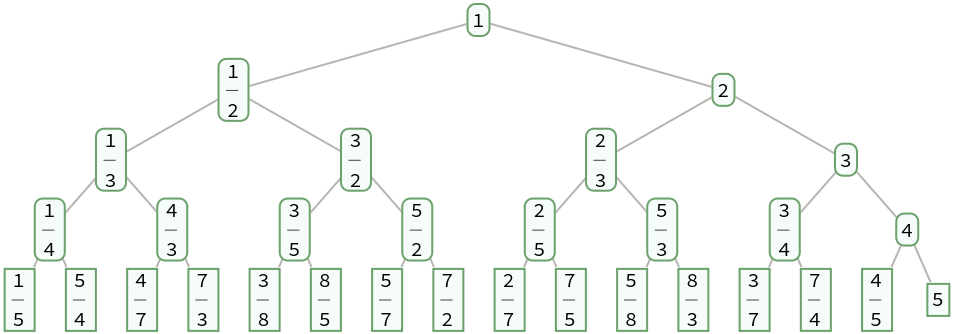In:=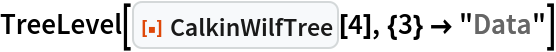Out=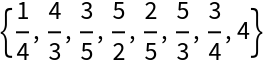In:=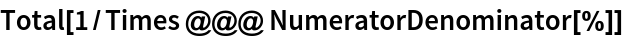Out=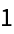Define a function that permutes the positions on level d by reversing the corresponding binary integers with d digits:

 In:=Permute the fractions on each level of a Calkin-Wilf tree:

 In:=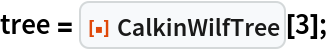In:=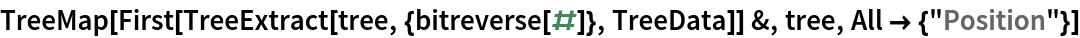Out=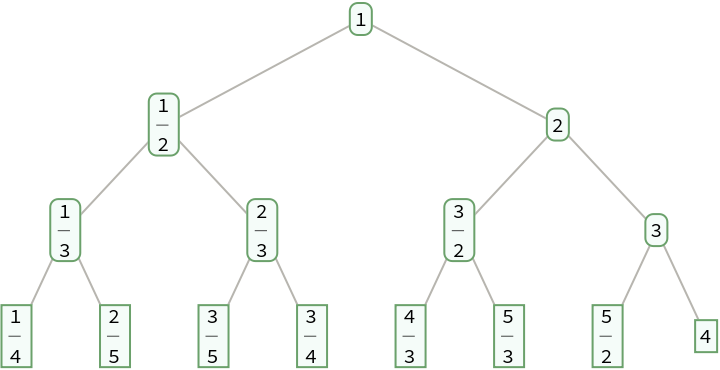The result is a Stern-Brocot tree:

 In:=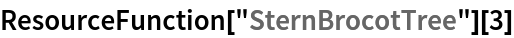Out=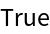## Version History

• 1.0.0 – 06 January 2023